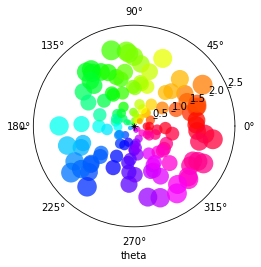# Polar Scatter Demo#

URL: http://matplotlib.org/examples/pie_and_polar_charts/polar_scatter_demo.html

```import numpy as np
import holoviews as hv
from holoviews import dim

hv.extension('matplotlib')
```## Define data#

```# Compute areas and colors
N = 150
r = 2 * np.random.rand(N)
theta = 2 * np.pi * np.random.rand(N)
colors = theta

scatter = hv.Scatter((theta, r), 'theta', 'r').redim(r=dict(range=(0,2.5)))
```

## Plot#

```scatter.opts(
alpha=0.75, color='theta', cmap='hsv', projection='polar', s=dim('r')*200)
```This web page was generated from a Jupyter notebook and not all interactivity will work on this website. Right click to download and run locally for full Python-backed interactivity.